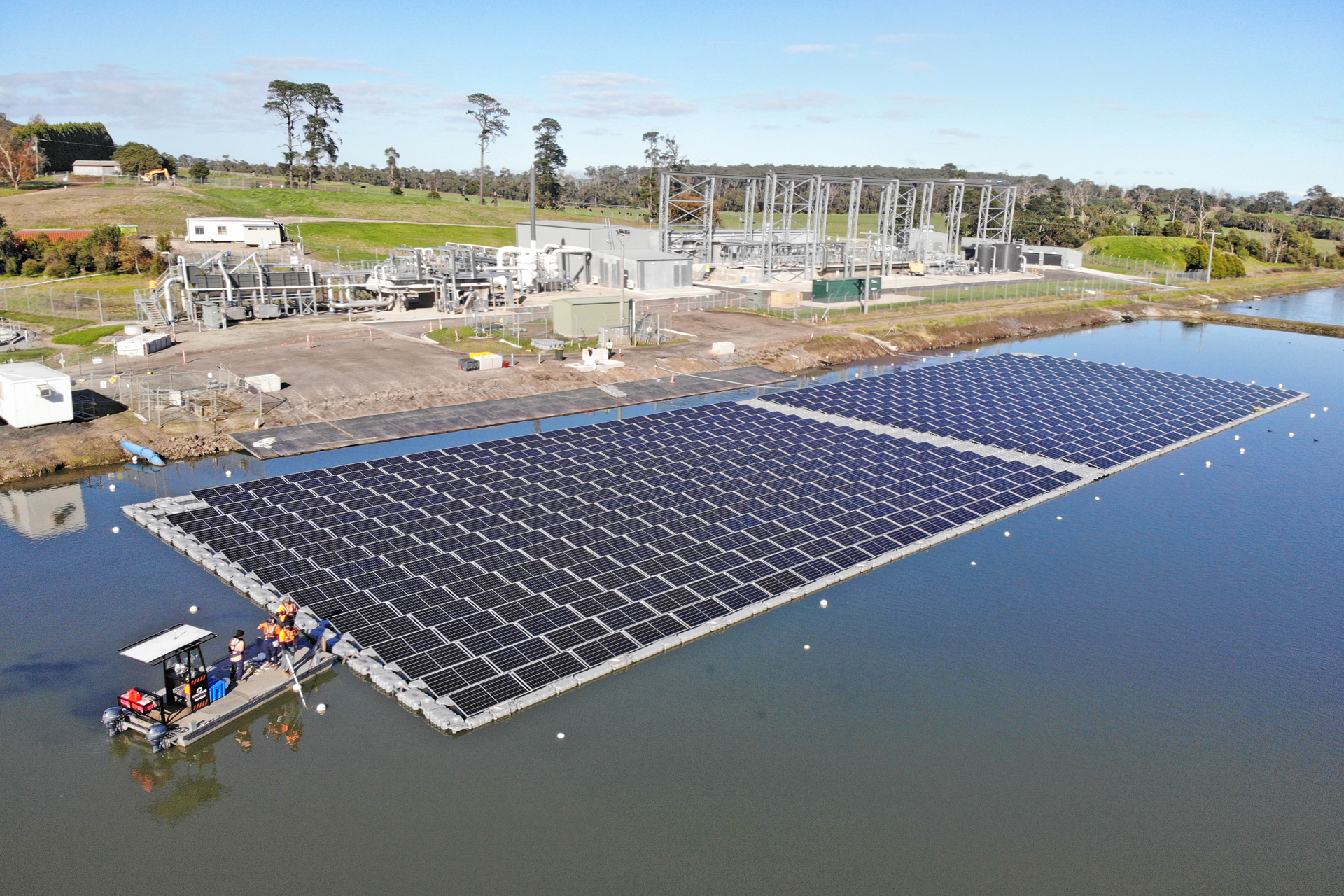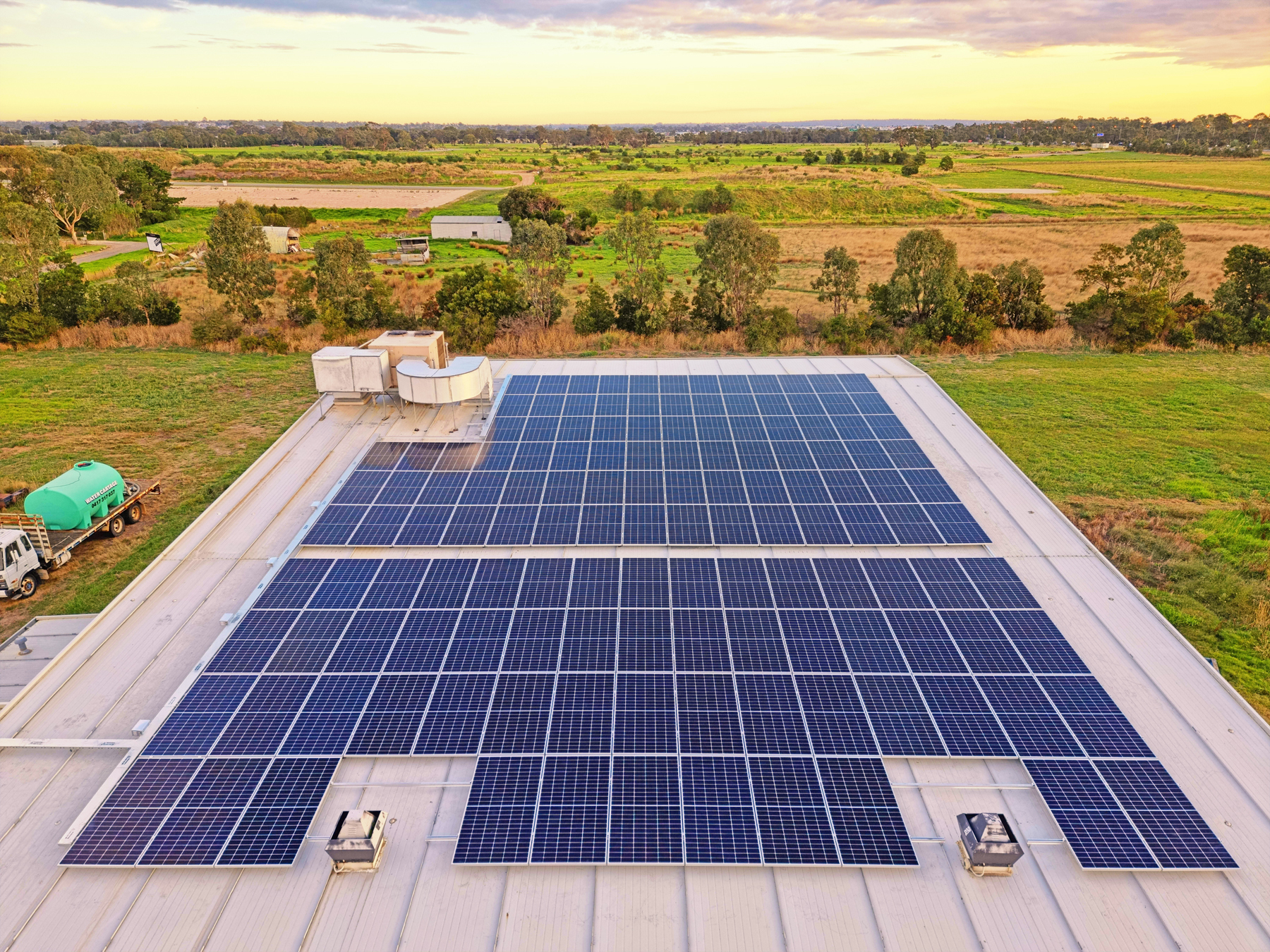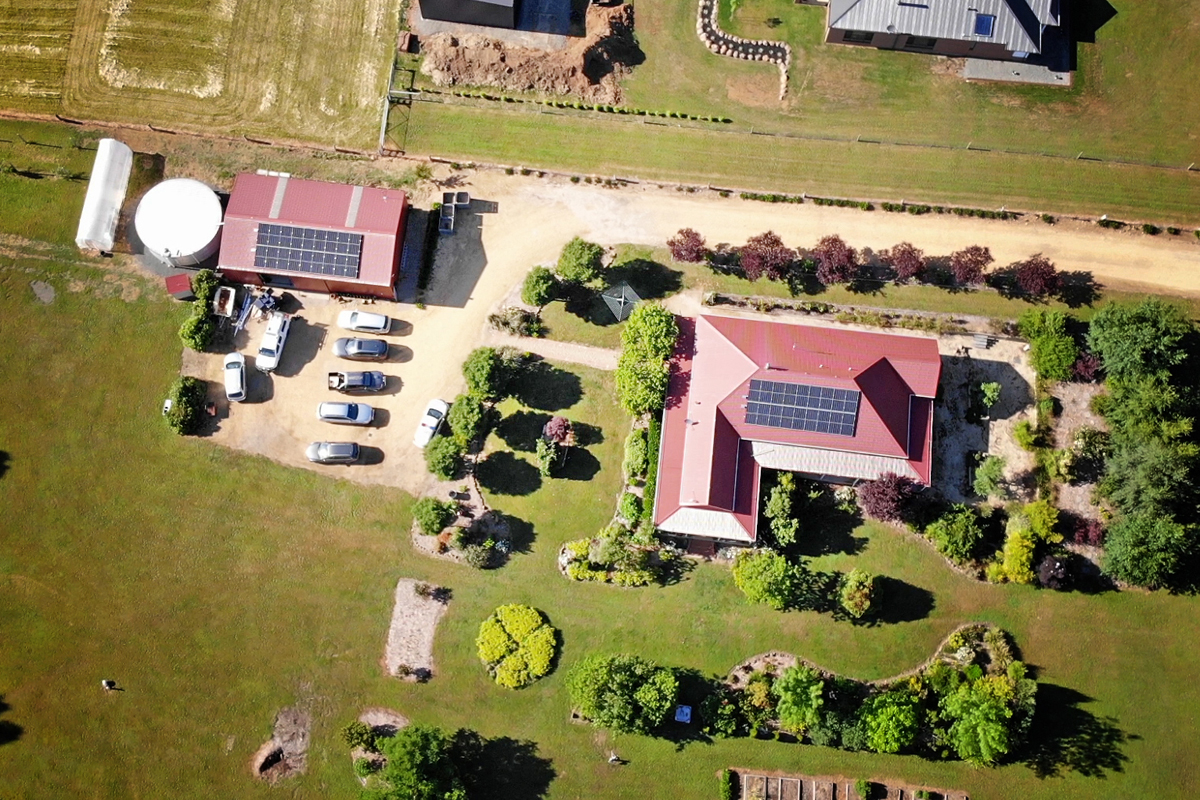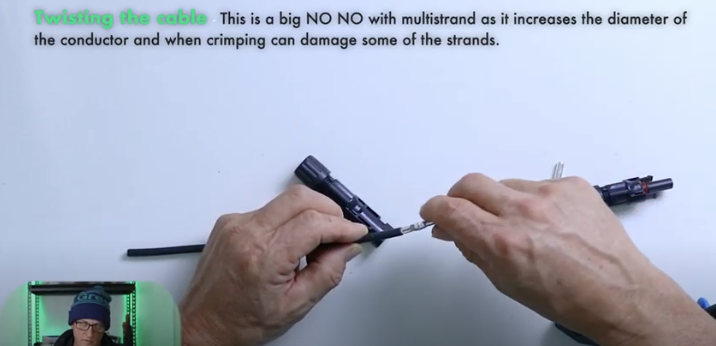## How much rail do we need?

Every solar project requires the use of framing or rail to support the panels so structural integrity is maintained, the manufacturer’s warranty is abided by and the system is safe. How many rail lengths are needed; how many mid and end clamps; how many  rail joiners and rail feet are questions every designer and operations manager asks.

In our first look at framing from a spreadsheet perspective the example given was a basic single row of panels with no calculations concerning the number of feet required.

This blog will discuss a more advanced  approach compared to the previous blog. The spreadsheet program referenced is Excel for MAC but any spreadsheet software can be used.

The format follows an input and output approach and as can be seen in the image above, there is a Solar panel Framing Inputs table with the cells in yellow signifying user input.

The first input is the row number panel brand, followed by the model, length of the panel, the width and the number of panels. These inputs are referenced in formulas that derive the outputs. The question Is this one string, Y or N can determine the number of DC isolators used and the question asking Is row separate determines the number of end clamps used.

With solar panel framing the main components are the frame or rail, top and bottom, mid clamps, end clamps, frame rail joiners and feet.

As can be seen from the above there are some additional questions from our first blog/video presentation that include the cantilever amount and the feet spacing, both in mm.

Now if the inputs for row 1 will be replicated in additional rows then the formula can be dragged down and then adjusted accordingly.

## Now for the outputs

As with the basic framing spreadsheet we discussed last time there are outputs for the number of mid and end clamps, frame joiners and DC isolators.

We will not look at these in detail but we will concentrate on the outputs concerning the number of panels, total kW capacity of the system, total number of frames/rail needed, the number of feet and the physical area taken up by the panels themselves.

## Total number of panels and output

This is a fairly simple calculation. It sums all the inputted numbers in cells E6:E16. Now if there are more than 11 rows just adjust the calculation to include them.

The total kW of the system is calculated from the total number of panels x by the wattage and in this case results in a total kW of system of 83.6 kW.

## Number of frames/rail needed

When calculating the total number of rails required each row is calculated and the result is rounded up to the nearest whole number and it relies on the calculated length of the frame, in this case 19610 mm and the rail length, in M6 (4000 mm) is divided into the total bottom frame length then the results in the two columns are summed =SUM(T6:T16+U6:U16)

## Number of feet needed

This calculation relies on the inputted values for the amount of cantilever over the last foot, both ends and the feet spacing in mm. The numbers for these inputs are 350 mm and 1000 mm respectively.

It also looks at the length of rail required, bottom rail as the extra amount for the DC isolator is not taken into consideration

The calculation is =(ROUNDUP((S6-(2*O6))/P6,0)+1)*2 and looks at the number in S6 which is 19610 mm and then subtracts from this 2 x O6, which is the cantilever amount, in this case of 350 mm for both ends.

Then the feet spacing amount, in this case 1000 mm is divided into the first figure, then rounded up, one is then added and the entire figure is multiplied by 2 as we are talking both top and bottom rails.

## Total area

Now we can look at what total surface area the panels take up...

… We use the top rail as reference as it takes up more room due to the additional rail required for the DC isolator so the calculation takes into account the sum of the rail lengths of each row then divides by 1000mm to get a figure in metres.

Then we multiply by the length and width of each panel used per row. It assumes that the same panel dimensions are used for each row. What the calculation does not do is to calculate the spacing between the rows. To do so would require an additional user input and we will be looking at this in a future video.

## Conclusion

The above example follows on from the basic framing spreadsheet video and includes how to calculate the number of feet required for a project with multiple rows and, of course, the number of mid and end clamps, framing.

The spreadsheet concepts are fairly basic but they give an understanding of how the calculations are derived and it’s really important from a costing and design perspective to get things right as the wrong calculations can end up eroding your gross margins.

Looking forward to presenting another spreadsheet based blog in the coming weeks - we've been getting some great feedback from designers about these so we've got a few more in the works.# Related posts for youCommercial
October 26, 2023

#### Greenwoood nominated for CEC solar awardsCommercial
September 6, 2022

#### Commercial solar for a fish farmCommercial
August 9, 2022

#### Trentham solar & battery: revisitedCommercial
July 26, 2022

#### Ground Mount and AgrivoltaicsCommercial
July 20, 2022

#### Solar and batteries; life in the MountainsTraining
June 1, 2022

#### MC4 connections: how to crimp correctly